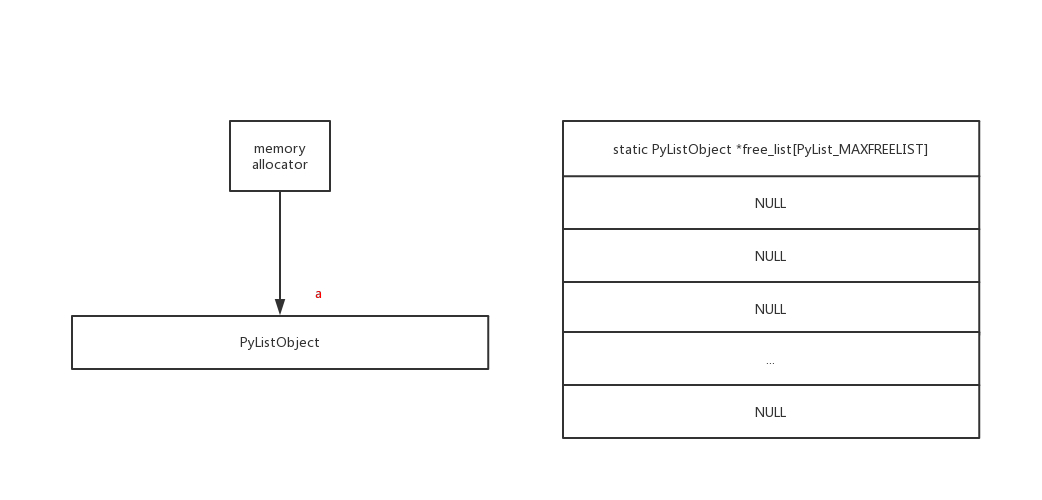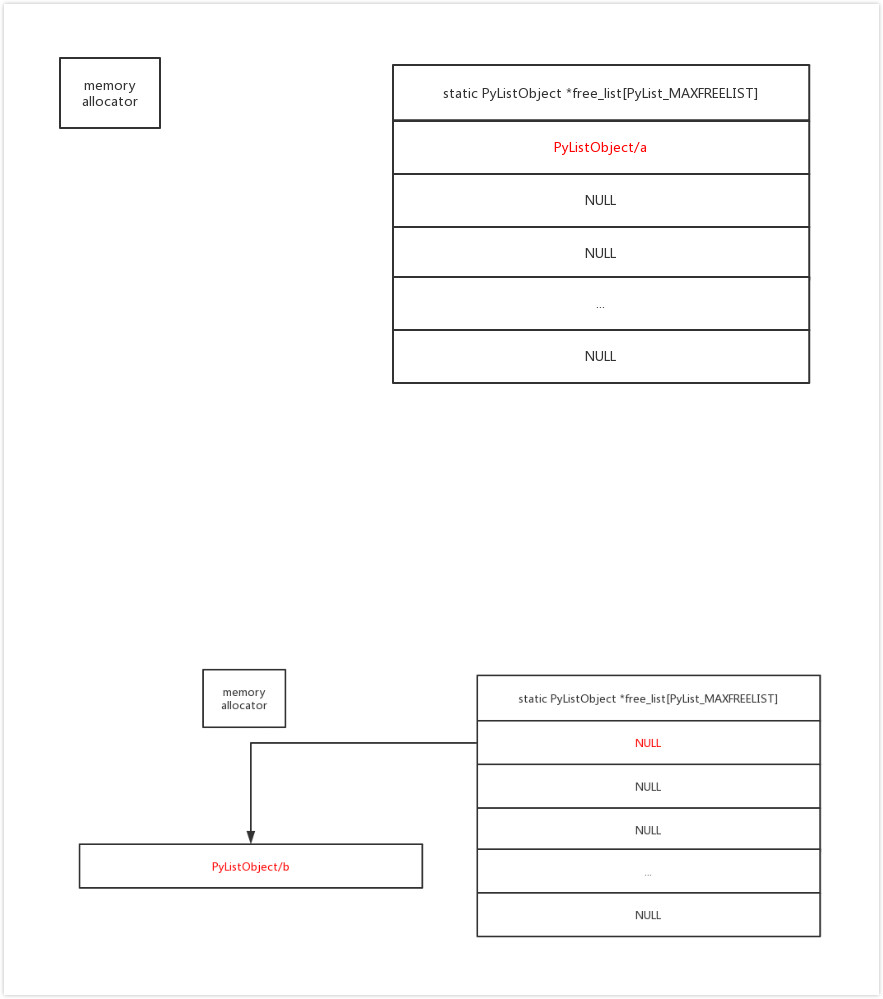## Python 为了提升性能，竟运用了共享经济

``````# 共用内存地址的例子
a = 100
b = 100
s = "python_cat"
t = "python_cat"

id(a) == id(b) # 结果：True
id(s) == id(t) # 结果：True``````

## 1、不可变对象的共享经济

``````# 空对象的差别
a = []
b = []
c = ()
d = ()

print(id(a)==id(b))  # 结果：False
print(id(c)==id(d))  # 结果：True``````

• 除了空元组，还有什么样的元组是“特权种族”？（PS：从元素的数量、类型、元素自身的大小考虑，就我小范围试验，还没发现。所以，空元组是独特的唯一？）
• 编译期与运行期有所区别，这在之前写字符串的 intern 机制时（《Intern机制的软肋》）也分析过。（PS：print(id([]) == id([]))，结果为 True，与上例先赋值再比较不同。）

## 2、可变对象的共享经济

``````# 实验版本：Python 3.6.1
a = [[] for i in range(4)]
print(id(a))

for i in range(len(a)):
print(f'{i} -- {id(a[i])}')
# a[i] = 1 # PS：可去除注释，再执行一次，结果的顺序有差别

del a
print("after del")

b = [[] for i in range(4)]
print(id(b))

for i in range(len(b)):
print(f'{i} -- {id(b[i])}')``````

``````2012909395656
0 -- 2012909395272
1 -- 2012909406472
2 -- 2012909395208
3 -- 2012909395144
after del
2012909395656
0 -- 2012909395272
1 -- 2012909406472
2 -- 2012909395208
3 -- 2012909395144``````Python 解释器在实现这个机制时，使用了一个叫做`free_list` 的全局变量，其工作原理是：

• 当创建新的对象时，则检查 free_list 内是否有可用对象，有则取出使用，没有则创建
• 当这些对象被析构时，则检查 free_list 是否有剩余空间，有则存入其中
• 某类对象存入 free_list 时，只保留“躯壳”，而清空其内部所有的元素（即只共享杯子，不共享杯中物）Python 解释器在内存管理上真是煞费苦心啊，在那些司空见惯的基本对象上，它施加了诸多的小魔法，在我们毫不觉察的时候，它们有条不紊地运作，而当我们终于见识清楚后，就不得不感叹它的精妙了。

Python 算得上是一个精打细算的“经济学家”了。

• 较小的数字、较短的字符串、布尔值与空元组等不可变对象，它们存在着“共享经济”的机制，提升了内存的使用效率
• 列表、集合与字典等可变对象，它们存在着预分配及超额分配等“供需平衡”的机制，提升了内存的分配效率
• 列表等对象还存在着共享“容器外壳”的机制，循环利用空闲资源，综合提升程序性能

PS：本文写作过半时，我觉得应该把它写入“喵星来客”系列，但思前想后，最终作罢了（主要是懒）。它们的思辨力及洞察力是一脉相承的，若你喜欢本文的话，我推荐阅读“喵星来客”系列（其中两篇）：

Python对象的身份迷思：从全体公民到万物皆数

Python对象的空间边界：独善其身与开放包容